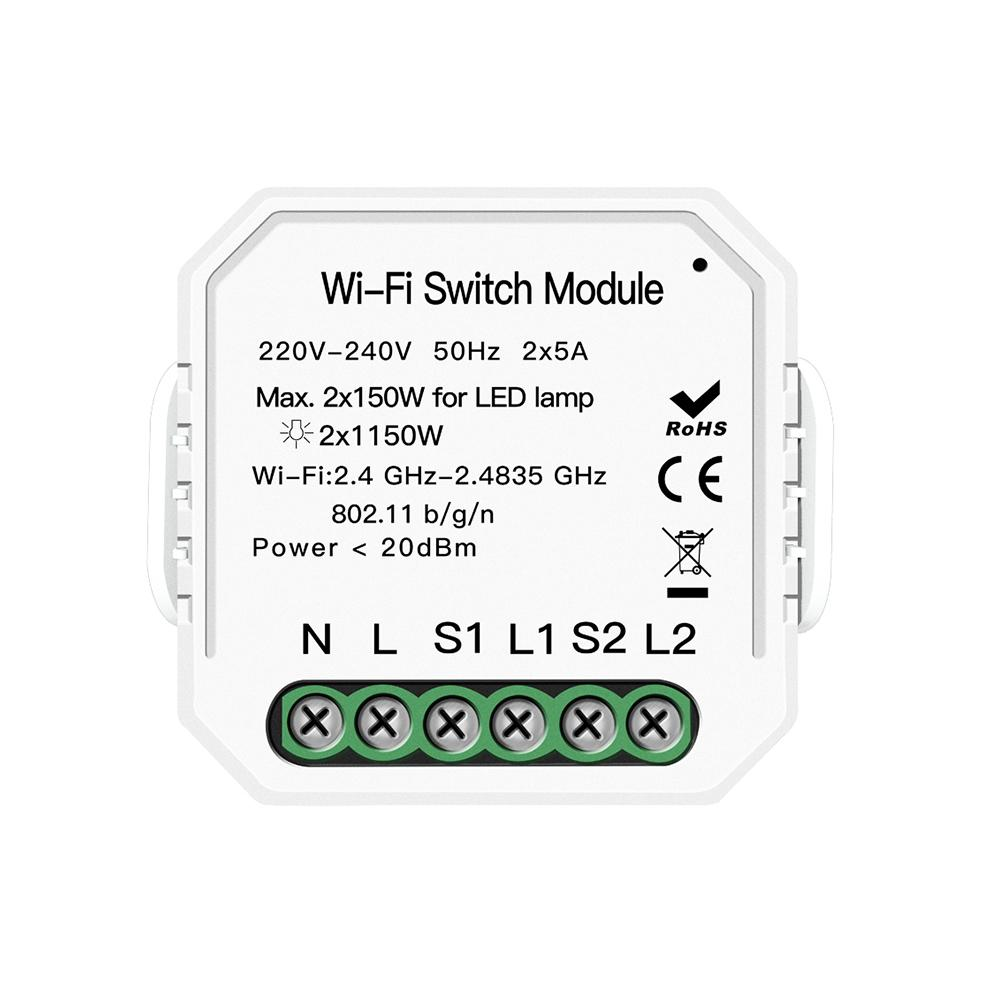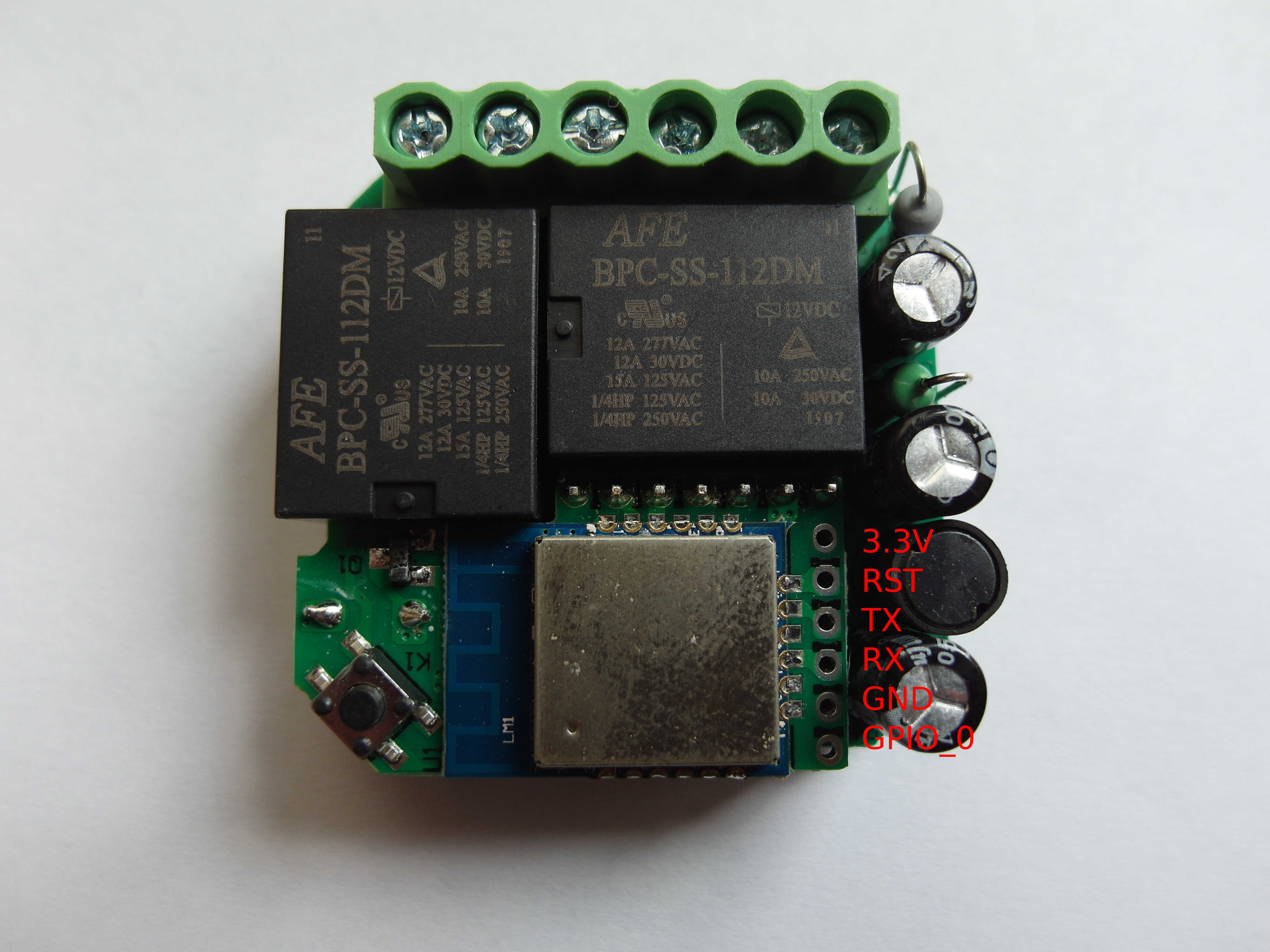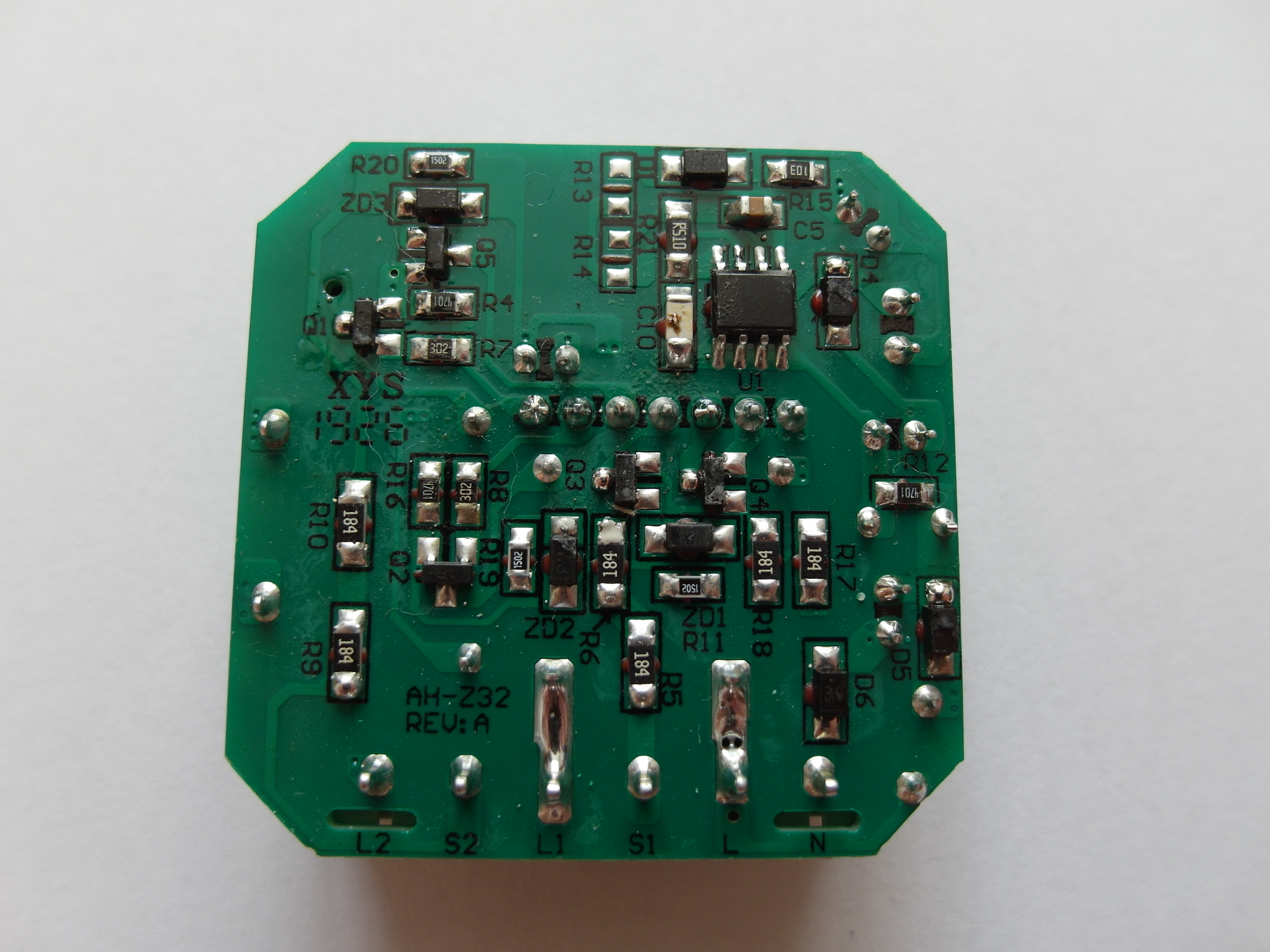Moes Switch Module (MS-104B)Available from:

Manufacturer:

GPIO #Component
GPIO00 None
GPIO01 None
GPIO02 Button1
GPIO03 None
GPIO04 Buzzer
GPIO05 None
GPIO09 None
GPIO10 None
GPIO12 Switch2
GPIO13 Switch1
GPIO14 Relay1
GPIO15 Relay2
GPIO16 None
FLAG None
Configuration (old format, will be converted to new template when applied)
``{"NAME":"Moes MS-104B","GPIO":[0,0,17,0,160,0,0,0,10,9,21,22,0],"FLAG":0,"BASE":18}``
Use code `BLAKADDER` when buying from moeshouse.com store for a 20% discount.

Some devices labelled Moes MS-104 are using a wi-fi chip unsupported by Tasmota.

This device uses AC frequency detection circuits on switch inputs supported since Tasmota 8.4.

After applying the template enable AC frequency detection feature using ‘SwitchDebounce’ command. ‘SwitchDebounce 69’ is a good place to start, which means 3 pulses (60 milliseconds) to switch on or off. When this feature is enabled, you can use them as regular switches (set ‘SwitchMode’, use in rules and do anything you can do with a regular switch).

# Deprecated way of handling switching

Use on Tasmota versions 8.3.1 and lesser

Use template

``````'{"NAME":"Moes MS-104B","GPIO":[0,0,17,0,160,0,0,0,43,42,21,22,0],"FLAG":0,"BASE":18}'
``````

Scripting is not supported with precompiled binary (at least 8.3) so you have to compile your own fw or download it (tasmota-scripting.bin) from tasmota/binaries. After that, you will have an option to add a script in Tasmota GUI: Configuration/Edit Script Below are scripts which do something like switchmode4, switchmode1 and switchmode0. The script for switchmode4 is created by @thxthx0, switchmode1 @caccia78 and @cHunter789, switchmode0 @antonio-fiol

SwitchMode1

``````>D
sw1=0
sw2=0
cnt1=0
cnt2=0
pwr1=0
pwr2=0

>B
=>print "WiFi - 2 relay / 2 switch"
;sleep 250 because counters are sleep sensitive
->sleep 250
>F
; Always reset counters - consider only counts in last 100ms
cnt1=pc
->Counter1 0

cnt2=pc
->Counter2 0

if cnt1>3
then
; counter1 has increased in last 100ms - check with >2 for debounce
sw1=1
else
; no counter incres --> switch is off
sw1=0
endif

if cnt2>3
then
; counter2 has increased in last 100ms - check with >2 for debounce
sw2=1
else
; no counter increas --> switch is off
sw2=0
endif

; turn on the light if switches on
if sw1==1
and pwr1==0
then
=>Power1 1
pwr1=1
endif

if sw2==1
and pwr2==0
then
=>Power2 1
pwr2=1
endif

;turn off the light if switches off
if sw1==0
and pwr1==1
then
=>Power1 0
pwr1=0
endif

if sw2==0
and pwr2==1
then
=>Power2 0
pwr2=0
endif

``````

SwitchMode4

``````>D
sw1=0
sw2=0
cnt1=0
cnt2=0
timer1=0
timer2=0
toggle1=0
toggle2=0

>B
=>print ""WiFi 2-Gang Switch Script""

>F
; Counter1/2 and Relay1/2 configured in template
cnt1=pc
cnt2=pc

if chg[cnt1]>0
then
; counter1 has changed, switch is on
sw1=1
else
; no change switch is off
sw1=0
endif

if chg[cnt2]>0
then
; counter2 has changed, switch is on
sw2=1
else
; no change switch is off
sw2=0
endif

; 100 ms timer
timer1+=1
timer2+=1

if sw1==0
and timer1>2
and timer1<30
then
;=>print short press1
toggle1^=1
=>Power1 %toggle1%
endif

if sw1==0
then
timer1=0
endif

if sw2==0
and timer2>2
and timer2<30
then
;=>print short press2
toggle2^=1
=>Power2 %toggle2%
endif

if sw2==0
then
timer2=0
endif

>S
; restart the device every sunday at 04:00
; to avoid possible issues when increasing counters and variables

if wday==1
and hours==4
and mins==0
and secs==0
then
->Restart 1
endif

``````

SwitchMode 0

``````>D
sw1=0
sw2=0
osw1=0
osw2=0
M:cnt1=0
M:cnt2=0
np=0

>B
=>print ""WiFi 2-Gang Switch Script""

>F
; Counter1/2 and Relay1/2 configured in template
cnt1=pc
cnt2=pc
cnt1=pc
cnt2=pc
cnt1=pc
cnt2=pc
->Counter1 0
->Counter2 0

if cnt1>2.5
then
; counter1 has changed, switch is on
sw1=1
else
; no change switch is off
sw1=0
endif

if cnt2>2.5
then
; counter2 has changed, switch is on
sw2=1
else
; no change switch is off
sw2=0
endif

;=>print %cnt1% %sw1% %osw1% %pwr%

if sw1!=osw1
then
osw1=sw1
if pwr==0
then
np=1
else
np=0
endif
=>Power1 %np%
=>print Power1 %np%
endif

if sw2!=osw2
then
osw2=sw2
if pwr==0
then
np=1
else
np=0
endif
=>Power2 %np%
=>print Power2 %np%
endif

>S
; restart the device every sunday at 04:00
; to avoid possible issues when increasing counters and variables

if wday==1
and hours==4
and mins==0
and secs==0
then
->Restart 1
endif

``````